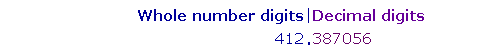S k i l l
i n
A R I T H M E T I C

Lesson 3

THE MEANING OF DECIMALS

A decimal -- 0.3865 -- is a proper fraction, which is a number less than 1. It is a part of number 1. To understand what that means we must introduce the ordinal numbers: first, second, third, fourth, and so on. The ordinal numbers express division into equal parts.

The counting-numbers have two forms:  cardinal and ordinal.

 1. What are the names of the cardinal numbers? One, two, three, four, and so on.

A cardinal number answers the question How many?

 2. What are the names of the ordinal numbers? First, second, third, fourth, and so on.

An ordinal number answers the question Which one?

We will now see that the ordinal numbers express division into equal parts.  An ordinal number names which part -- the third part, the fourth, the fifth, and so on.

Division into equal partsIf we divide 15, for example, into three equal parts -- into three 5's -- then we say that 5 is the third part of 15. We say that because 15 is the third multiple of 5:  5, 10, 15.  We use that same ordinal number to name the part.

If we divide a quantity into four equal parts, then we call each part a fourth; if into five equal parts, a fifth; if into one hundred equal parts, a hundredth; but if into two equal parts, we say half.

And so with the exception of half, an ordinal number indicates into how many equal parts a quantity has been divided.

A third, a fourth, a fifth, and so on, are the names of parts. Those names belong to language itself, not just to mathematics. Those ordinals are prior to the names of numbers we call fractions, which, we are about to see, are numbers we need for measuring, and are the parts of number 1.

We will go into this more in Lesson 15.

The decimal system

Since our numbering system is based on the powers of 10, it is called a decimal system. Decem in Latin means ten.  In the previous Lessons we learned about whole numbers, which are the repeated additions of 1:
1, 2, 3, 4, and so on.  Here we will learn about numbers that are less than 1. They are the numbers we create when we divide 1 into equal parts -- where 1 is now a continuous unit.  And since this is a decimal system, those parts of 1 will have the ordinal names of the powers of 10:  tenths, hundredths, thousandths, and so on.

First, we will divide One into ten equal parts, and so each part is called a Tenth.If we divide each Tenth into ten equal parts, then One will be in one hundred equal parts -- count themAnd so we have divided One into Hundredths.  If we divide each Hundredth into ten equal parts, then each tiny piece will be a Thousandth part of One.  And so on.

Those Tenths, Hundredths, Thousandths are called the decimal units.

 3. Which numbers are the decimal units? They are the units less than 1 -- they are the parts of 1 -- named as tenths, hundredths, thousandths, ten-thousandths, and so on.

The sequence of names of the decimal units follows the same sequence as the powers of 10.  They are those powers in their ordinal form.

Whole units Decimal units
ten tenth
hundred hundredth
thousand thousandth
ten-thousand ten-thousandth
hundred-thousand      hundred-thousandth
million millionth

 4. What is the function of the decimal point?The decimal point separates the whole number on the left from the decimal digits on the right. In fact, it was originally called the separatrix, the separator. Each decimal digit then indicates the number of tenths, hundredths, thousandths, and so on.

412.387056

The decimal point signifies "and," in the sense of  "plus."

That is the whole number 412 plus 3 tenths of 1, plus 8 hundredths of 1, plus 7 thousandths; and so on.

Any number written with a decimal point is loosely called a decimal. A number less than 1 -- a proper fraction -- written as a decimal, we call a decimal fraction. A number written with a numerator and denominator we call a common fraction (Lesson 20).

The positions to the right of the decimal point are called the decimal

412.387056

places; the 1st, the 2nd, the 3rd, and so on.  Each decimal digit occupies a decimal place.

 5. Which decimal unit is at each decimal place?The digit at the 1st decimal place shows the number of Tenths.  In the number above, there are 3 tenths.

The 2nd decimal place shows the number of Hundredths.  In that number, there are 8 hundredths.  And so on.

The first digit to the left of the decimal point -- the 2 -- shows the number of Ones.

As with whole numbers, each digit has its place value:

2.3875. . .

Ones, tenths, hundredths, thousandths, ten-thousandths, . . .

As with the whole numbers, each place is ten times the place to its right.

One is ten tenths.

One-tenth is ten hundredths.

One-hundredth is ten thousandths.

One-thousandth is ten ten-thousandths.

And so on.

Example 1.  In this number

534.267

a)  there are how many ones?

Answer.   4.  The ones place is the first digit to the left of the decimal point.

b)  How many hundredths?

Answer.   6.  Hundredths (ordinal) is a decimal unit.  It falls to the right of the decimal point.

c)  How many hundreds?

Answer.   5.  Hundred (cardinal) is a whole unit.  It falls to the left of the decimal point.

Example 2.  Expanded form.   Just as the numeral for every whole number stands for a sum (Lesson 2), so does the numeral for every decimal. Here is the expanded form of

534.267.

534.267 = 5 Hundreds + 3 Tens + 4 Ones + 2 Tenths + 6 Hundredths

7 Thousandths.

At this point, please "turn" the page and do some Problems.

or

Continue on to Section 2.